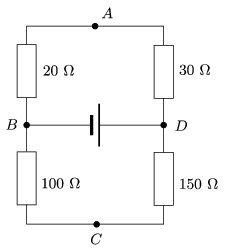Mathematical and Physical Journal
for High Schools
Issued by the MATFUND Foundation
 Already signed up? New to KöMaL?

#Problem P. 4175. (September 2009)

P. 4175. Which of the points, marked by A, B, C, D, of the circuit shown in the figure, are at the same potential. Between which two points is the potential difference the greatest?(3 pont)

Deadline expired on October 12, 2009.

Sorry, the solution is available only in Hungarian. Google translation

Megoldás. $\displaystyle A$ és $\displaystyle C$ azonos potenciálú. $\displaystyle B$ és $\displaystyle D$ között van a legnagyobb feszültség.

### Statistics:

 169 students sent a solution. 3 points: 114 students. 2 points: 17 students. 1 point: 26 students. 0 point: 11 students. Unfair, not evaluated: 1 solutions.

Problems in Physics of KöMaL, September 2009